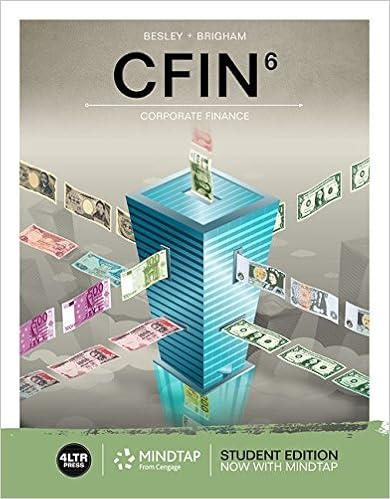# Which one of the following terms applies to a bond

• Notes
• PrivateBraveryDeer8003
• 96
• 100% (14) 14 out of 14 people found this document helpful

This preview shows page 5 - 9 out of 96 pages.

##### We have textbook solutions for you!
The document you are viewing contains questions related to this textbook.The document you are viewing contains questions related to this textbook.
Chapter 6 / Exercise 6-4
CFIN
BesleyExpert Verified
19. Which one of the following terms applies to a bond that initially sells at a deep discount and pays no interest payments? A. Callable B. Income C. Zero coupon D. Convertible E. Tax-free
20. The price at which a dealer will purchase a bond is called the _____ price.
6-5
##### We have textbook solutions for you!
The document you are viewing contains questions related to this textbook.The document you are viewing contains questions related to this textbook.
Chapter 6 / Exercise 6-4
CFIN
BesleyExpert Verified
Chapter 06 - Interest Rates and Bond Valuation 21. The price at which an investor can purchase a bond from a dealer is called the _____ price.
22. A bond trader just purchased and resold a bond. The amount of profit earned by the trader from this purchase and resale is referred to as the:
23. Which one of the following is the quoted price of a bond? A. Par value B. Discount price C. Face value D. Dirty price E. Clean price
24. Which one of the following is the price that an investor pays to purchase an outstanding bond?
6-6
Chapter 06 - Interest Rates and Bond Valuation 25. A real rate of return is defined as a rate that has been adjusted for which one of the following?
26. Which one of the following is the rate of return an investor earns on a bond before adjusting for inflation?
27. Which one of the following refers to the relationship between nominal returns, real returns, and inflation? A. Call premium B. Fisher effect C. Conversion ratio D. Bid-ask spread E. Clean-dirty spread
28. The term structure of interest rates represents the relationship between which of the following?
6-7
Chapter 06 - Interest Rates and Bond Valuation 29. The inflation premium:
30. Changes in interest rates affect bond prices. Which one of the following compensates bond investors for this risk?
•••